# Age of family

The age of father and son is in the ratio 10: 3. The age of the father and daughter is in a ratio of 5: 2. How old are a father and a son if the daughter is 20?

o =  50
s =  15

### Step-by-step explanation: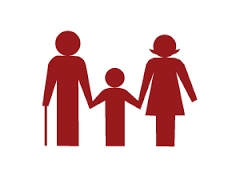Did you find an error or inaccuracy? Feel free to write us. Thank you!Tips to related online calculators
Need help to calculate sum, simplify or multiply fractions? Try our fraction calculator.
Check out our ratio calculator.
Do you want to convert time units like minutes to seconds?

## Related math problems and questions:

• Family and age ratiosThe age of father and son are in the ratio 10:3; the age of father and daughter are in the ratio 5:2. How old are father and son if the daughter is 20 years old? In what ratio is age sister and brother?
• Father and daughterFather is 36 years old, daughter is 20 years less. What will be the ratio between them when they are 10 years more?
• AgesFather is 36 years old, and his daughter is 4 years old. Write down the ratio of the age of father and daughter. In what ratio will the ages of father and daughter after 4 years?
• Father and son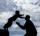Father and son are together 78 years. Son is 25 years younger than father. How old is the son?
• Father and sonFather is 48 years old, son 23. When will the father be twice older as a son?
• Large familyThe average age of all family members (children, mother, father, grandmother, grandfather) is 29 years. The average age of parents is 40 years, grandparents 66 years and all children are 5 years. How many children are there in this family?
• What is 9What is the value of x in the proportion 2 and one-fourth over x = 1 and one-half over 3 and three-fifths? 2 and two-fifths 5 and two-fifths 8 and 1 over 10 12 and 3 over 20
• Mother and daughter 2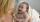The mother is 40 years older than her daughter. How old is the mother if her age is eight thirds age of daughter?
• The familyHow old is a mother if she is four times older than her daughter and 5 years ago she was even seven times older than her daughter? Today, a son is 30 years younger than a father. 7 years ago, a father was seven times older than a son. How old is a son tod
• The fatherThe father is 3 times older than his son. 10 years ago, a father was 7 times older than his son. How old are father and son?
• Age ratioJanko is 14 years old. The age ratio of Janka and Zuzka is 2: 3. What was the ratio seven years ago?
• Ratio of volumesIf the heights of two cylindrical drums are in the ratio 7:8 and their base radii are in the ratio 4:3. What is the ratio of their volumes?
• The father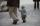The father is 36 years old. The son is 6 years old. How many years will a father be 3 times older than a son?
• Daughters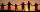The man conducting the census asks a woman to age of three daughters. Woman says when multiply the age getnumber 72; if their ages add up, get a number of our house, as you see. The man says: That is not enough to calculate their ages. She says: my oldest
• Father and son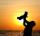Father is three times older than his son. Twelve years ago, the father was nine times more senior than the son. How old are father and son?
• Interior anglesCalculate the interior angles of a triangle that are in the ratio 2: 3: 4.
• Marriage sttusIn our city, there are 3/5 of the women married to 2/3 of the men. Find what part of the population is free.